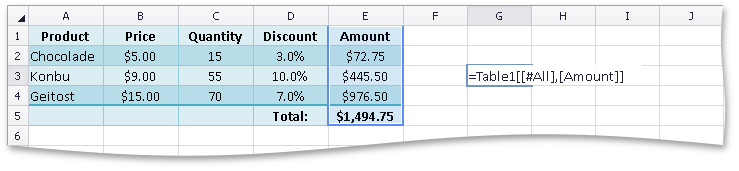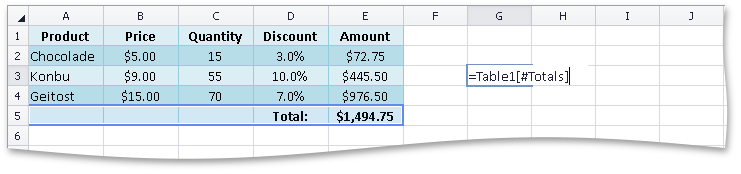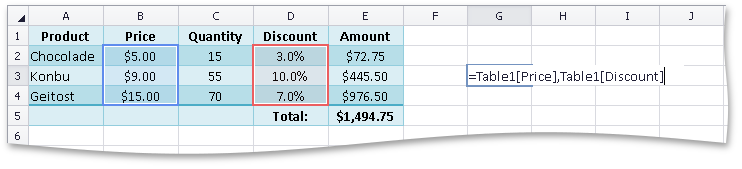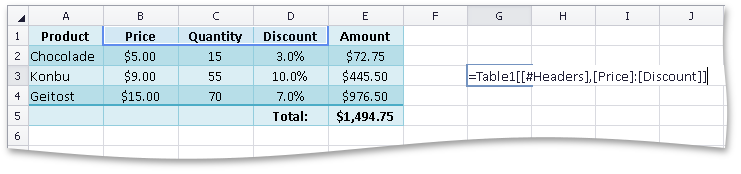# Cell Referencing

A cell reference is a set of coordinates that specify the position of a cell or cell range on a worksheet. Use cell references in formulas to obtain and process data contained in the corresponding cells. The results of formulas that use cell references are automatically updated each time the values of these cells are changed.

SpreadsheetControl supports the following cell reference types.

A1 Cell References
This is the default cell reference style. A cell is referred to by a column letter and row number.
R1C1 Cell References
When this cell reference style is enabled, both rows and columns are numbered on a worksheet. A cell is referred to by a row number preceded by “R” and a column number preceded by “C”.
Cross-Worksheet Cell References
References to cells located in other worksheets.
3D Cell References
References to the same cells located on multiple worksheets within a workbook.
External Cell References
References to cells located in other workbooks.
Structured Cell References
References to tables and table data ranges.

Refer to the following articles for more information on how to use cell references in formulas or to access a cell:

## A1 Cell References

The A1 cell reference style is used by default. The A1 cell reference is a combination of column and row headings to which the cell belongs - a column letter is followed by a row number. By default, column and row headings are displayed at the top and at the left of a worksheet (see How to: Show and Hide Row and Column Headings).

The following table provides examples of A1 references to a single cell, and various cell ranges (including references to entire rows and columns).

Cell Reference Description
E7 Refers to a single cell located at the intersection of column E and row 7.
A2:B5 Refers to a range that includes cells from the top left cell A2 to the bottom right cell B5.
B:B Refers to a range that includes the entire column B.
B:H Refers to a range that includes the entire columns from B to H.
5:5 Refers to a range that includes the entire row 5.
5:10 Refers to a range that includes the entire rows from 5 to 10.

When used in a formula, a cell reference can be relative, absolute or mixed depending on whether or not it should be automatically adjusted when the formula is copied.

### Relative Cell References

A relative cell reference in a formula is based on the relative position of a referenced cell and a cell containing a formula. To keep this relative position unchanged, the cell reference is automatically changed each time you copy a formula to another cell. For example, if you copy a formula with a relative reference to cell B2 from cell C3 to D6, the reference will automatically change from B2 to C5.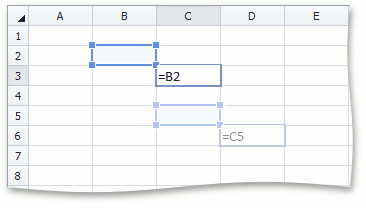### Absolute Cell References

An absolute cell reference in a formula always refers to a specific cell, and it does not change if the formula is copied or moved to another cell. In absolute cell references, the column letter and row number are preceded by the ‘\$’ sign. For example, if you copy a formula with an absolute reference to cell B2 from cell C3 to D6, the reference will remain unchanged (\$B\$2).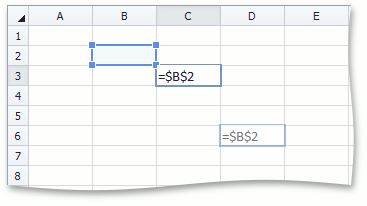### Mixed Cell References

A mixed cell reference in a formula can either be combined from an absolute reference to a cell column and a relative reference to a cell row (for example, \$B2), or from a relative reference to a cell column and an absolute reference to a cell row (for example, A\$1). If the formula is copied, the absolute element of the mixed reference (the column letter or row number preceded by the ‘\$’ sign) will remain unchanged, and the relative element of the reference will automatically be adjusted.

For example, if you copy a formula with the \$C3 mixed reference from cell B2 to D6, the reference will change to \$C7.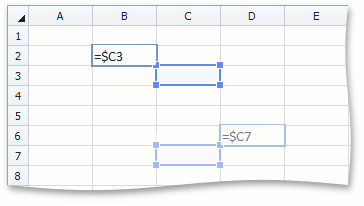## R1C1 Cell References

You can also use the R1C1 reference style, where both rows and columns are numbered in a worksheet. To switch on this style in a workbook, use the DocumentSettings.R1C1ReferenceStyle property. A cell reference of the R1C1 style is a combination of “R” followed by the row number and “C” followed by the column number.

Cell Reference Description
R5C7 Refers to a single cell located at the intersection of row 5 and column 7.
R3C4:R7C10 Refers to a range that includes cells from the top left cell R3C4 to the bottom right cell R7C10.
C4 Refers to a range that includes the entire column 4.
C5:C7 Refers to a range that includes the entire columns from 5 to 7.
R10 Refers to a range that includes the entire row 10.
R12:R15 Refers to a range that includes the entire rows from 12 to 15.

Like the A1 cell reference style, the R1C1 cell reference style also supports the relative, absolute and mixed reference types.

### Relative Cell References

In relative R1C1 cell references, the row and column numbers are enclosed in square brackets. Positive numbers refer to cells below and/or to the right, relative to a cell containing a formula. Negative numbers refer to cells above and/or to the left, relative to the cell containing the formula.

If an R1C1 cell reference does not include a row or column number, it refers to the cell in the same row or column that contains the cell with the formula.

When you copy a formula with relative cell references in the R1C1 style, the notation of these cell references remains the same in copied formulas, while the A1 relative references are changed for each copied formula to keep the relative position of the referenced cell and the cell containing the formula.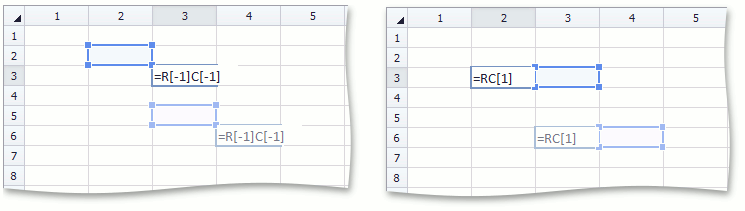### Absolute Cell References

In absolute R1C1 cell references, row and column numbers are used without brackets.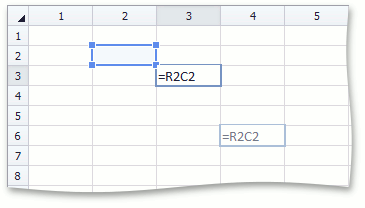### Mixed Cell References

An R1C1 cell reference can either be combined from an absolute reference to a cell row and a relative reference to a cell column (for example, R10C[-5]), or from a relative reference to a cell row and an absolute reference to a cell column (for example, RC5).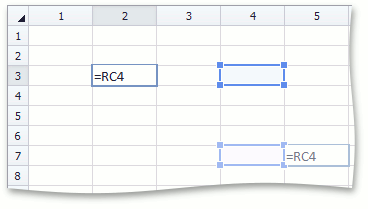Note

If the R1C1 cell reference style is switched on in a workbook, A1 references cannot be used in formulas, and vice-versa. The style of cell references used in formulas is automatically adjusted when you change the active cell reference style in the workbook.

## Cross-Worksheet Cell References

In formulas, you can use references to cells located in other worksheets. To do this, specify the worksheet name before the cell reference, and separate them by an exclamation point (!).

When using a worksheet name in a cell reference, enclose it in single quotation marks (‘) in the following cases.

• The worksheet name contains a character other than a letter or number (spaces, parentheses, braces, etc.).
• The worksheet name starts with neither a letter nor an underscore symbol (“_”).
• The worksheet name is the same as an A1 cell reference (“A1”, “\$M\$15”, etc.).
• The worksheet name starts with an R1C1 cell reference notation.

If you rename a worksheet, this worksheet name is automatically updated in all cell references where it is used.

``````// Sum values of cells located in different worksheets.
workbook.Worksheets["Sheet1"].Cells["H15"].Formula = "= Sheet2!C3 + Sheet3!C5";
``````

## 3D Cell References

3D references allow you to process data contained in the same cells on multiple worksheets within a workbook. To create a 3D reference, specify the range of worksheet names before the cell (or cell range) reference, and separate them by an exclamation point (!).

For example, the formula in the following image sums all values in cells B2 through C3 located on worksheets Sheet2, Sheet3, Sheet4 and Sheet5. If you insert other worksheets between Sheet2 and Sheet5 (for example, by creating new worksheets, or by duplicating or moving existing worksheets), the B2:C3 cell ranges of the added worksheets will be included into the calculation. If you remove worksheets from the Sheet2:Sheet5 worksheet range, the values of these worksheets are excluded from the calculation.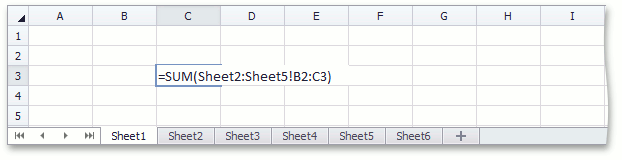## External Cell References

A reference that refers to a cell, cell range or defined name in another workbook is called an external reference. In order to use external references, all referenced workbooks should be included in the IWorkbook.ExternalWorkbooks collection of the workbook that contains the external references.

Note

Creating external workbooks with circular references between each other is not recommended. In this case, you can get incorrect calculation results, since our internal calculation engine does not trace dependencies between external workbook cells.

An external reference includes the entire path to the workbook file, the workbook file name in square brackets ([ ]), the worksheet name, an exclamation point (!), and the cell reference.

This example demonstrates how to programmatically create an external reference in a workbook loaded into one SpreadsheetControl instance to the B3 cell in the Book1.xlsx workbook loaded into another SpreadsheetControl.

``````using DevExpress.Spreadsheet;
// ...

// Access the current workbook where you wish to use external references.
Worksheet worksheet = workbook.Worksheets;

// Access the source workbook that contains the cells to be referred to from the current workbook.

// Add the source workbook to the collection of external workbooks for the current workbook.

// Create an external reference in the current workbook.
worksheet["C3"].Formula = "='c:\\Temp\\[Book1.xlsx]Sheet1'!B3";
``````

The ExternalWorkbookCollection.Add method with the alias parameter allows you to specify a custom workbook name that you can use in external references instead of the original file name or when the external workbook is not saved to a file. In this case, an external reference includes the specified workbook name in square brackets ([ ]), the worksheet name, an exclamation point (!) and the cell reference.

This example demonstrates how to create an external reference in a workbook loaded into one SpreadsheetControl instance to cells located in another workbook in a second SpreadsheetControl.

``````using DevExpress.Spreadsheet;
// ...

// Access the current workbook where you wish to use external references.
Worksheet worksheet = workbook.Worksheets;

// Access the source workbook that contains cells to be referred to from the current workbook.

// Add the source workbook to the collection of external workbooks
// and specify a custom name that can be used in external references instead of the file name.

// Create an external reference in the current workbook.
worksheet["C3"].Formula = "=[MyExternalWorkbook]Sheet1!B3";
``````

An external reference to a defined name in another workbook includes the source workbook name, an exclamation point (!) and the defined name. For example, =book.xlsx!range_name.

If the scope of the defined name is a worksheet in another workbook, specify this worksheet name in an external reference. For example, =[book.xlsx]Sheet3!range_name.

## Structured Cell References

Structured references allow you to refer to tables and different ranges within tables. A structured reference has the following syntax.

=TableName[[#Data],[ColumnName]]

• TableName is a table name (Table.Name).
• [#Data] is a special item specifier that refers to a data range of a table or table column (as in the current example). You can also use the following special item specifiers in structured references, to refer to specific parts of tables or table columns: [#All], [#Headers], [#Totals] and [#This Row].
• [ColumnName] is a table column specifier (TableColumn.Name). If it is not preceded by any special item specifier, the table column specifier refers to the column data range (excluding the column header and total cells).

To combine column specifiers in structured references, you can use the following operators: colon - to refer to a range of two or more adjacent columns, comma - to refer to a combination of two or more columns, and space - to refer to an intersection of two or more columns.

The images below illustrate examples of structured references using different special item specifiers and reference operators.